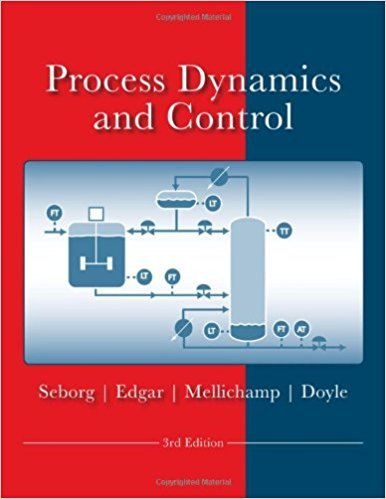×
Get Full Access to Process Dynamics And Control - 3 Edition - Chapter 11 - Problem 11.24
Get Full Access to Process Dynamics And Control - 3 Edition - Chapter 11 - Problem 11.24

×

# A control system has Gv = Gm = 1 and a second-orderISBN: 9780470128671 148

## Solution for problem 11.24 Chapter 11

Process Dynamics and Control | 3rd Edition

• Textbook Solutions
• 2901 Step-by-step solutions solved by professors and subject experts
• Get 24/7 help from StudySoup virtual teaching assistantsProcess Dynamics and Control | 3rd Edition

4 5 1 291 Reviews
28
0
Problem 11.24

A control system has Gv = Gm = 1 and a second-order process Gp with Kp = 2, r 1 = 4 min, and r 2 = 1 min, which is to be controlled by a PI controller with Kc = 2 and r1 = r 1 = 4 min (i.e., the integral time of the controller is set equal to the dominant time constant). For a set-point change (a) Determine the closed-loop transfer function. (b) Derive the characteristic equation, which is a quadratic polynomial ins. Is it overdamped or underdamped? (c) Can a large value of Kc make the closed-loop process unstable?

Step-by-Step Solution:

Problem 11.24A control system has G = v = 1mand a second-order process transfer function with K = 2, p 1= 4 min, and 2 2 = 1 min, which is to be controlled by a PI controller with K = c and 1 = 4(i.e., the integral time of the controller is set equal to the dominant time constant). For aset-point change (a) Determine the closed-loop transfer function. (b) Derive the characteristicequation, which is a quadratic polynomial ins. Is it overdamped or underdamped (c) Can alarge value of Kc make the closed-loop process unstable Step-by-step solution Step 1 of 8 ^(a)Consider the following open-loop process transfer function: K p G = …………(1) p (1s + 1)( 2 + 1)Here,The process gain is K apTime constants are 1 and .2Consider that process gain K isp and time constants 1 and 2 are 4 and 1 respectively.Substitute the available values in equation (1). 2 Gp (4s + 1)(s + 1) ………(2)

Step 2 of 8

Step 3 of 8

##### ISBN: 9780470128671

This textbook survival guide was created for the textbook: Process Dynamics and Control, edition: 3. This full solution covers the following key subjects: min, closed, process, set, Loop. This expansive textbook survival guide covers 24 chapters, and 420 solutions. Since the solution to 11.24 from 11 chapter was answered, more than 1099 students have viewed the full step-by-step answer. The full step-by-step solution to problem: 11.24 from chapter: 11 was answered by , our top Engineering and Tech solution expert on 11/15/17, 04:03PM. The answer to “A control system has Gv = Gm = 1 and a second-order process Gp with Kp = 2, r 1 = 4 min, and r 2 = 1 min, which is to be controlled by a PI controller with Kc = 2 and r1 = r 1 = 4 min (i.e., the integral time of the controller is set equal to the dominant time constant). For a set-point change (a) Determine the closed-loop transfer function. (b) Derive the characteristic equation, which is a quadratic polynomial ins. Is it overdamped or underdamped? (c) Can a large value of Kc make the closed-loop process unstable?” is broken down into a number of easy to follow steps, and 103 words. Process Dynamics and Control was written by and is associated to the ISBN: 9780470128671.

Unlock Textbook Solution